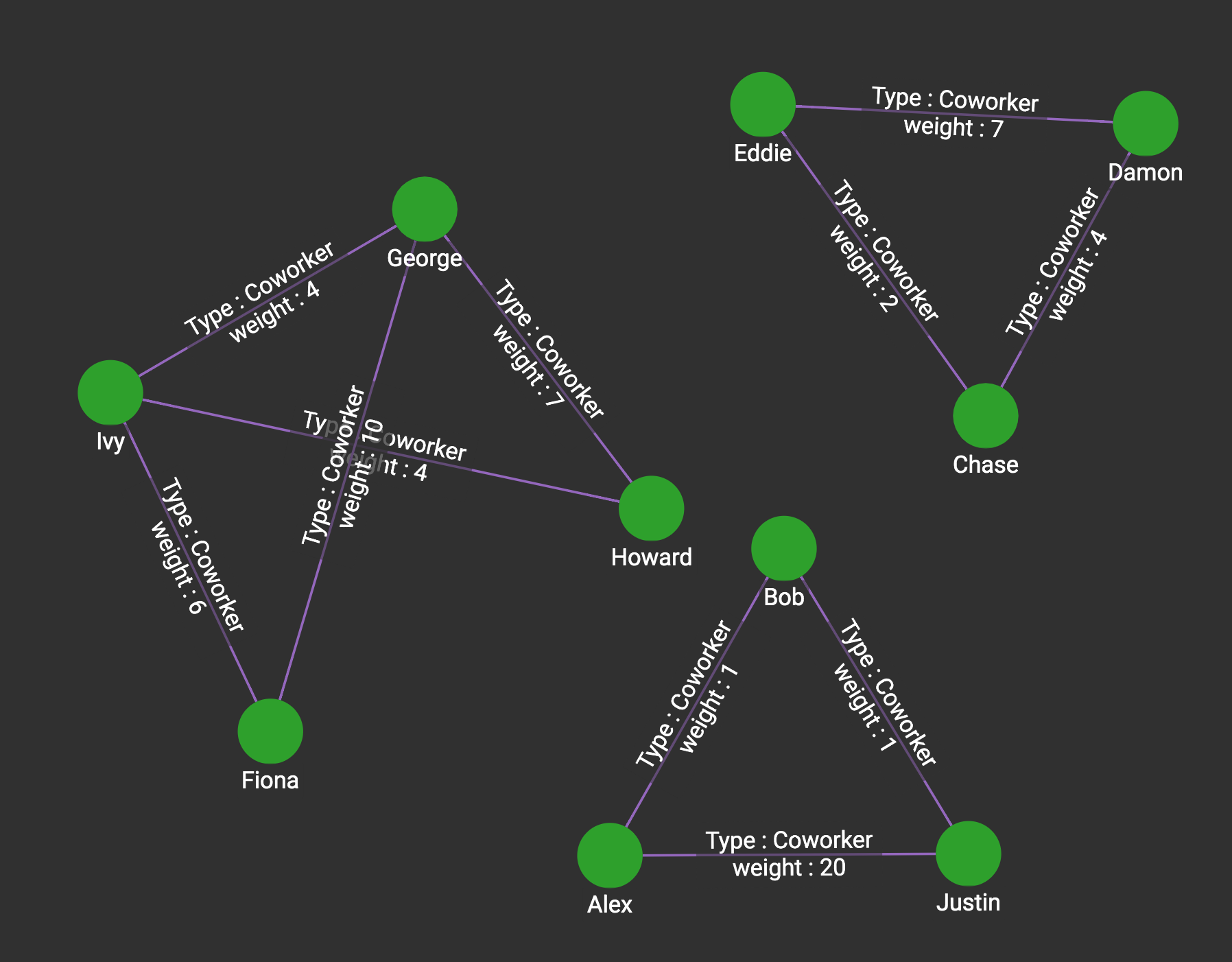# Maximal Independent Set

An independent set of vertices does not contain any pair of vertices that are neighbors, i.e., ones which have an edge between them. A maximal independent set is the largest independent set that contains those vertices; you cannot improve upon it unless you start over with a different independent set. However, the search for the largest possible independent set (the maximum independent set, as opposed to the maximal independent set) is an NP-hard problem: there is no known algorithm that can find that answer in polynomial time. So we settle for the maximal independent set.

This algorithm finds use in applications wanting to find the most efficient configuration which "covers" all the necessary cases. For example, it has been used to optimize delivery or transit routes, where each vertex is one transit segment and each edge connects two segments that can NOT be covered by the same vehicle.

## Specifications

``````tg_maximal_indep_set(STRING v_type, STRING e_type,
INT max_iter = 100, BOOL print_accum = TRUE, STRING file_path = "")``````
Characteristic Value

Result

A set of vertices that form a maximal independent set.

Input Parameters

• `STRING v_type`: Name of vertex type to use

• `STRING e_type`: Name of edge type to use

• `INT max_iter`: maximum number of iterations for the search

• `BOOL print_accum`: If True, output JSON to standard output

• `STRING file_path`: If not empty, write output to this file.

Result Size

Size of the MIS: unknown. Worst case: If the graph is a set of N unconnected vertices, then the MIS is all N vertices.

Time Complexity

O(E), E = number of edges

Graph Types

Undirected edges

## Example

Consider our social10 graph, with three components.It is clear that for each of the two triangles — (Alex, Bob, Justin) and (Chase, Damon, Eddie) — we can select one vertex from each triangle to be part of the MIS. For the 4-vertex component (Fiona, George, Howard, Ivy), it is less clear what will happen. If the algorithm selects either George or Ivy, then no other independent vertices remain in the component. However, the algorithm could select both Fiona and Howard; they are independent of one another.

This demonstrates the uncertainty of the Maximal Independent Set algorithm and how it differs from Maximum Independent Set. A maximum independent set algorithm would always select Fiona and Howard, plus 2 others, for a total of 4 vertices. The maximal independent set algorithm relies on chance. It could return either 3 or 4 vertices.